# Sliding motion along an inclined plane

(diff) ← Older revision | Latest revision (diff) | Newer revision → (diff)
This article discusses a scenario/arrangement whose statics/dynamics/kinematics can be understood using the ideas of classical mechanics.
View other mechanics scenarios

The scenario here is a dry block (with a stable surface of contact) on a dry fixed inclined plane, with$\theta$ being the angle of inclination with the horizontal axis.

The extremes are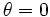$\theta = 0$ (whence, the plane is horizontal) and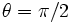$\theta = \pi/2$ (whence, the plane is vertical).

## Basic components of force diagram

A good way of understanding the force diagram is using the coordinate axes as the axis along the inclined plane and normal to the inclined plane. For simplicity, we will assume a two-dimensional situation, with no forces acting along the horizontal axis that is part of the inclined plane (in our pictorial representation, this no action axis is the axis perpendicular to the plane).

In this case, assuming a stable surface of contact, and that the inclined plane does not break under the weight of the block, and no other external forces, we get the following equation from Newton's first law of motion applied to the axis perpendicular to the inclined plane:$N = mg \cos \theta$

where$N$ is the normal force between the block and the inclined plane,$m$ is the mass of the block, and$g$ is the acceleration due to gravity.$N$ acts inward on the inclined plane and outward on the block. In particular, note that:

• When$\theta = 0$,$N = mg$. This is the usual idea that the normal force exerted on a horizontal surface equals the mass times the acceleration due to gravity, which we customarily call the weight.
• When$\theta = \pi/2$,$N = 0$. This indicates that the block and the inclined plane are barely in contact and hardly pressed together.
• As$\theta$ increases from$0$ to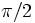$\pi/2$, the normal force, and hence, the extent to which the block and the inclined plane are pressed together, reduces.

For simplicity, we ignore the cases$\theta = 0$ and$\theta = \pi/2$ unless specifically dealing with them.

For the axis down the inclined plane, the gravitational force component is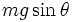$mg\sin \theta$. The magnitude and direction of the friction force are not clear. We have three cases. If the block is sliding down the inclined plane, then we get, from Newton's second law of motion:$ma = mg \sin \theta - \mu_kN$

where$a$ is acceleration measured positive down the inclined plane. If the block is sliding up the inclined plane, then we get, from Newton's second law of motion:$ma = mg \sin \theta + \mu_kN$

where$a$ is acceleration measured positive up the inclined plane. Finally, if the block is stationary, we get:$mg \sin \theta \le \mu_sN$

The three cases simplify, respectively, to:

•$a = g (\sin \theta - \mu_k\cos \theta) = g \cos \theta (\tan \theta - \mu_k)$
•$a = g (\sin \theta + \mu_k \cos \theta) = g \cos \theta (\tan \theta + \mu_k)$
•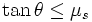$\tan \theta \le \mu_s$.

## Behavior with given initial dynamics

### Initially placed at rest

If the block is initially placed gently at rest, we have the following cases:

•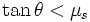$\tan \theta < \mu_s$, or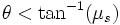$\theta < \tan^{-1}(\mu_s)$: In this case, the body does not start sliding down, and instead stays stable where it is. The magnitude of static friction is Failed to parse (unknown function "\mg"): \mg \sin \theta , and its direction is upward along the inclined plane, precisely balancing and hence canceling the component of gravitational force along the inclined plane.
•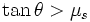$\tan \theta > \mu_s$ or$\theta > \tan^{-1}(\mu_s)$: In this case, the body starts sliding down, and the downward acceleration is given by$a = g(\sin \theta - \mu_k \cos \theta) = g \cos \theta (\tan \theta - \mu_k)$.

The angle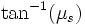$\tan^{-1}(\mu_s)$ is termed the angle of repose, since this is the largest angle at which the block does not start sliding down.

### Given an initial speed downward

If the block is given an initial downward speed, the acceleration is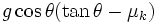$g \cos \theta (\tan \theta - \mu_k)$ downward, and its long-term behavior is determined by whether$\tan \theta < \mu_k$ or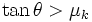$\tan \theta > \mu_k$:

• If$\tan \theta < \mu_k$, then the block's speed reduces with time. The magnitude of retardation is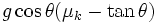$g \cos \theta (\mu_k - \tan \theta)$. If the incline is long enough, this retardation continues until the block reaches a speed of zero, at which point it comes to rest and thence stays at rest.
• If$\tan \theta > \mu_k$, then the block's speed increases with time. The magnitude of acceleration is$g \cos \theta (\tan \theta - \mu_k)$. The block thus does not come to a stop and keeps going faster as it goes down the incline.

### Given an initial speed upward

If the block is given an initial upward speed, the acceleration is$g \cos theta (\tan \theta + \mu_k)$ downward, i.e., the retardation is$g \cos \theta(\tan \theta + \mu_k)$. We again consider two cases:

• If$\tan \theta < \mu_k$, then block's speed reduces with time as it rises. If the incline is long enough, the block eventually comes to a halt and stays still after that point.
• If$\tan \theta > \mu_s$, the block's speed reduces with time as it rises, until it comes to a halt, after which it starts sliding downward, just as in the case of a block initially placed at rest.
• If$\tan \theta$ is between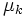$\mu_k$ and$\mu_s$, the behavior is somewhat indeterminate, in the sense that whether the block starts sliding down after stopping its upward slide is unclear.